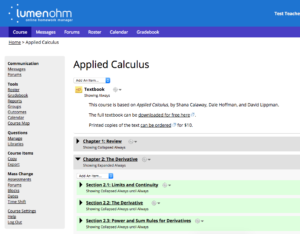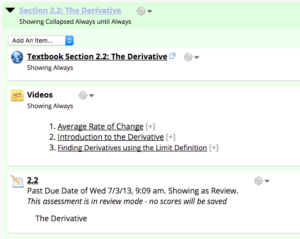## Content Overview

 Course Materials YES NO Online Homework Questions? X Editable Text? Video Support? X – in the OHM course Written Assessments/ Test? Workbook?

### Text

This course is based on Applied Calculus, by Shana Calaway, Dale Hoffman, and David Lippman.  The full textbook can be downloaded for free here. Students will learn to apply calculus in economic and business settings, like maximizing profit or minimizing average cost, finding elasticity of demand, or finding the present value of a continuous income stream.

### Topic Overview

Some of the differences between the content in this course and a calculus course for engineering, science or math majors is:

• No trigonometry
• The applications are focused on business, social, and life sciences
• Fewer theorems, no proofs
• Definite limits, no infinite limits

The course is delivered in Lumen OHM in 4 chapters that cover the following material

Chapter 1: Review

• Section 1: Functions
• Section 2: Operations on Functions
• Section 3: Linear Functions
• Section 4: Exponents
• Section 6: Polynomials and Rational Functions
• Section 7: Exponential Functions
• Section 8: Logarithmic Functions

Chapter 2: The Derivative

• Section 1: Limits and Continuity
• Section 2: The Derivative
• Section 3: Power and Sum Rules for Derivatives
• Section 4: Product and Quotient Rules
• Section 5: Chain Rule
• Section 6: Second Derivative and Concavity
• Section 7: Optimization
• Section 8: Curve Sketching
• Section 9: Applied Optimization
• Section 10: Other Applications
• Section 11: Implicit Differentiation and Related Rates

Chapter 3: The Integral

• Section 1: The Definite Integral
• Section 2: The Fundamental Theorem and Antidifferentiation
• Section 3: Antiderivatives of Formulas
• Section 4: Substitution
• Section 5: Additional Integration Techniques
• Section 6: Area, Volume, and Average Value
• Section 7: Applications to Business
• Section 8: Differential Equations

Chapter 4: Functions of Two Variables

• Section 1: Functions of Two Variables
• Section 2: Calculus of Functions of Two Variables
• Section 3: Optimization
• Table of Integrals

Length: One semester

Delivery: This course is delivered in Lumen OHM with automated grading of numerical and algebraic answers, similar to WebAssign and MyMathLab. This makes it appropriate for self-study by students, or as a face to face course. This course can be imported into your LMS (Blackboard, Canvas, Moodle) to provide a single-sign on experience for your students, as well as grade return for  you.

## Online Content

Lumen OHM provides randomized, algorithmically generated homework with automated grading of numerical and algebraic answers, similar to WebAssign and MyMathLab.

Each chapter in the course is divided into sections that contain a reading, relevant video examples, and an online homework set.